# AP Computer Science A : Counting Statement Executions

## Example Questions

### Example Question #25 : Program Analysis

public void countStatements() {

       int a = 10, b = 15, c = 7;

       // Start

       for(int i = 0; i < a; i += 2) {

              b--;

              System.out.print("*");

       }

       for(int i = b; i >=0; i--) {

              for(int j = 0; j < c; j++) {

                     System.out.print("*");

              }

       }

       // End

}

In the code above, how many times will System.out.print be called between the comments "// Start" and "// End"?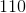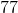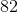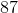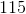Explanation:

Start by counting the number of times the first loop will cycle. Notice that the loop control variable (namely, i) is increased by 2 for each looping. This means that you will loop for i = 0, 2, 4, 6, 8. It will not loop for 10, for then, i will equal a. That will terminate the loop. Thus, you have five loopings thus far. Note, also that this means that b is decremented 5 times. Thus, at the end of this loop, b now equals 10.

For the second loop, you must be careful, for it has two loops. The outer loop will run from i = 10 to i = 0.  This is a total of 11 loopings. (This is important to note because it runs not only for 10 through 1 but also for the final number, 0. The loop within that loop will run from j = 0 to j = 6 (i.e. one less than c, which is 7). This is a total of 7 loopings. Therefore, these nested loops will run a total of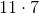or 77 times.  The total calls to System.out.print will be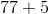, or 82.

### Example Question #1 : Counting Statement Executions

public void countStatements() {

       int[] array = new int;

       for(int i = 0; i < array.length; i++) {

              array[i] = 3 * i + 1;

              System.out.println("Yay!");

       }

       for(int i = 0; i < array; i++) {

              array[i]--;

              System.out.println("Yay!");

       }

       for(int i = array; i > 0; i--) {

              System.out.println("Yay!");

       }

}

How many calls will be made to System.out.println in the code above?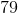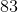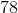Explanation:

Let us count for each loop, paying attention to variables as needed. The first loop will run a total of 54 times. Thus it will produce 54 total executions. Notice also how it initializes the array. This will be used in the second loop. Each element is equal to 3i + 1. Thus, we will have:

a = 1; a = 4; a = 7; a = 10; a = 13; a = 16; etc.

Now, loop two will use a in controlling its execution. Notice that it runs from i = 0 to i = 15. For each execution, it reduces the value of a[i] by one. Thus, be careful. It will eventually run for a, making it to be 15. This will then be used for the loop control. This means that it actually runs only from i = 0 to i = 14. Thus, the loop runs a total of 15 times.

The last loop runs from i = 9 to i = 1. (Note: This is because of the decrement in the second loop.) This is a total of 9 executions.

Thus, the total count of executions is: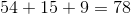### Example Question #27 : Program Analysis

How many statements are executed in the code snippet below?

for (int i = 0; i < arr.length; i++) {

if (i == 2) {

i++;

}

}

6

2

3

5

5

Explanation:

The statements executed in the code snippet are in order:

1. int i = 0
2. i < arr.length
3. i++ (which is equivalent to i = i + 1)
4. i == 2
5. i++

These statements are all executions that happen in order each time the for loop is run. Sometimes there may only be 4 executions if the if statement is false.